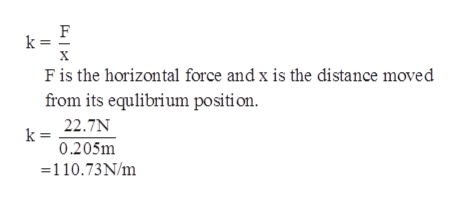# A 2.20 kg mass is attached to a spring and placed on a horizontal, smooth surface. A horizontal force of 22.7 N is required to hold the mass at rest when it is pulled 0.205 m from itsequilibrium position (the origin of the x axis). The mass is now released from rest with an initial displacement of xi = 0.205 m, and it subsequently undergoes simple harmonic oscillations. Calculate the speed when the displacement equals one third of the maximum value.Incorrect. Tries 4/6 Previous TriesSubmit AnswerCalculate the acceleration when the displacement equals one third of the maximum value.Tries 0/6Submit Answer

Question
25 viewshelp_outlineImage TranscriptioncloseA 2.20 kg mass is attached to a spring and placed on a horizontal, smooth surface. A horizontal force of 22.7 N is required to hold the mass at rest when it is pulled 0.205 m from its equilibrium position (the origin of the x axis). The mass is now released from rest with an initial displacement of xi = 0.205 m, and it subsequently undergoes simple harmonic oscillations. fullscreenhelp_outlineImage TranscriptioncloseCalculate the speed when the displacement equals one third of the maximum value. Incorrect. Tries 4/6 Previous Tries Submit Answer Calculate the acceleration when the displacement equals one third of the maximum value. Tries 0/6 Submit Answer fullscreen
check_circle

Step 1

The spring constant of the spring ishelp_outlineImage TranscriptioncloseF k = Fis the horizontal force and x is the distance moved from its equlibrium position 22.7N 0.205m =110.73N/m fullscreen
Step 2

The angular velocity is

Step 3

(a)

The speed when the displacement equals...

### Want to see the full answer?

See Solution

#### Want to see this answer and more?

Solutions are written by subject experts who are available 24/7. Questions are typically answered within 1 hour.*

See Solution
*Response times may vary by subject and question.
Tagged in

### Vibrations